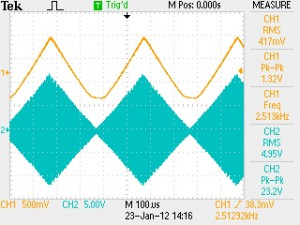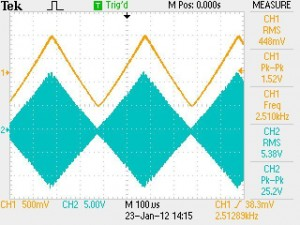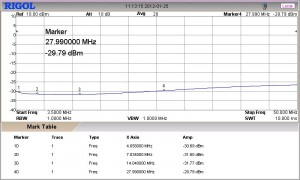# SMT & SMT-Pro Station monitor specifications verification measurementsFig. 1 Modulation envelope demodulation without the detector bias option.

Fig. 1 Note the clipping at low modulation levels (upper trace) especially evident during the modulation trough. This phenomena is caused by the inherent lack of the detector level bias current.Fig. 2 Modulation envelope derived from fully modulated RF signal with the optional detector bias current.

Fig. 2 Note the demodulated product (upper waveform) clearly shows the improvement at the trough modulation level resulting from the addition of the detector level bias current option.

Fig. 3 Note the RF envelope (top waveform), detected product (bottom waveform) without bias current option. This is caused by the intrinsic lack of detector bias current at the modulation trough.

Fig. 4 Note the lack of clipping at low modulation levels especially evident during the modulation trough. This is the result of adding low level detector bias current available on the SMT-Pro option.

Fig 5. Note the significant harmonic distortion (-24.4 db) levels resulting from modulation through clipping without the detector bias option. This is especially noticeable at low power level such QRP operation. At higher levels this effect is significantly reduced.

Fig. 6 Note the significantly reduced harmonic distortion levels resulting from eliminating modulation through clipping with the detector bias option. This is especially noticeable at low power level such QRP operation.  At higher  levels this effect is significantly reduced. The second harmonic distortion is down -31.2 dB.   At higher modulation levels of > 5watts, second order harmonic distortion is -45 dB.  All other modulation products are virtually eliminated.

Fig. 7 This graph shows the modulated RF envelope (lower trace) along with the demodulated signal (upper trace). Note that the transition times easily meet the bandwidth specifications (10-30,000 Hz) there is no spurious distortion ringing, overshoot present.Fig. 8 Sampler 2-50 mHZ bandwidth
Fig. 8 The nominal sampler output equals -30dB of the RF being sampled. This is a power ratio reduction of 1000. This also equals a voltage ratio reduction of 31.6228. Decibels state a power ratio, not an amount. They tell how many times more (positive dB) or less (negative dB) but not how much more or less in absolute terms. Decibels are logarithmic, not linear. For example, 20 dB is not twice the power ratio of 10 dB. The equation for decibels is A = 10*log10(P2/P1) (dB) where P1 is the power being measured, and P1 is the reference to which P2 is being compared. To convert from decibel measure back to power ratio: P2/P1 = 10^(A/10) Voltage is more easily measured than power, making it generally more convenient to use: A = 20*log10(V2/V1). The equation for obtaining voltage ratio from dB is V2/V1 = 10^(A/20). Thus, to obtain the equivalent voltage (Peak, P-P, or RMS) for -30dB multiply the sampled voltage times 31.63. For example if the RMS voltage at the sampler = 5 volts, than the actual RF RMS voltage at the sampler input would be 5×31.63=158.15. The power (P=E^2/R) would be 500.2 Watts.

Fig. 9 The wideband graph of the sampler output is shown here. While the nominal sampler output equals is -30dB of the RF being sampled, here at 4MHz it is -30.75dB, and 21.91 dB at 144.53 MHz.  Again, the equation for obtaining voltage ratio from dB is V2/V1 = 10^(A/20). Thus, -21 dB equals a voltage-ratio of 11.22 and the power ratio equals 125.89.  To obtain the equivalent voltage (Peak, P-P, or RMS) for -21 dB, multiply the sampled voltage times 11.22. For example, if the RMS voltage at the sampler = 5 volts, than the actual RF RMS voltage at the sampler input would be 5×11.22=56.1 The power (P=E^2/R) would be 62.9 Watts.

Fig. 10 Station monitor insertion loss without detector bias current option is show here. The insertion loss (-.03dB at HF frequencies) is barely measurable. This is the current consumed by the detector only (a few micro amps). For practical purposes this loss can be ignored since it equals a power radio of 1.00693, so again, virtually all the power passing through the sampler is delivered to the load.

Fig. 11  Station monitor insertion loss with detector bias current option is show here. The insertion loss (-.25dB at HF and 1dB at 200MHz) is barely measurable. This is the current consumed by the detector and its biasing current supply (a few micro amps). For practical purposes this loss can be ignored since it equals a power radio of 1.05925, so virtually all the power passing through the sampler is delivered to the load.

Fig. 12 The graph of specious emission of a typical linear amplifier (Ameritron AL811H) passing through the SMT & SMT-Pro station monitor. Note, the worst case harmonic spurious emission is -63.15 dB from the fundamental frequency of 14MHz. This is the residual spurious emission of the AL811H amplifier. Remarkably, when not overdriven, the AL811H despite its ancient design, exhibits excellent  specious emission characteristics. The additional spurious emissions contributed by station monitor measurements were negligible.  It should be noted that these spurious emission are at least 10 times (20dB) better than the minimum allowed by the  FCC: §97.307 Emission standards  (d) For transmitters installed after January 1, 2003, the mean power of any spurious emission from a station transmitter or external RF amplifier transmitting on a frequency below 30 MHz must be at least 43 dB below the mean power of the fundamental emission.

Back to products page